引用本文: 李娟, 张韵, 陈涛. 改进RRT算法在未知三维环境下AUV目标搜索中的应用 [J]. 智能系统学报, 2022, 17(2): 368-375.LI Juan, ZHANG Yun, CHEN Tao. Application of the improved RRT algorithm to AUV target search in an unknown 3D environment [J]. CAAI Transactions on Intelligent Systems, 2022, 17(2): 368-375.
 Citation: LI Juan, ZHANG Yun, CHEN Tao. Application of the improved RRT algorithm to AUV target search in an unknown 3D environment [J]. CAAI Transactions on Intelligent Systems, 2022, 17(2): 368-375.• 中图分类号: TP24

## Application of the improved RRT algorithm to AUV target search in an unknown 3D environment

• 摘要: 针对未知水下环境下的自主水下航行器（autonomous underwater vehicle，AUV）目标搜索问题，传统方法搜索速度慢且以解决二维平面下搜索问题为主，本文提出了一种基于改进RRT (rapid-exploration random tree)的未知三维环境目标搜索算法。在搜索方面，分别建立了包括目标存在概率地图、不确定度地图、区域遍历度地图在内的实时地图并设定其更新规则，根据搜索目标建立决策函数；在局部规划方面，将滚动规划与改进RRT算法相结合，规划出到搜索决策点的路径。二者的结合，实现了AUV在三维空间下在线实时搜索。仿真表明，该算法具有较强的遍历能力，提高了三维空间下目标搜索的速度。

Abstract: To solve the target search problem of autonomous underwater vehicles (AUV) in an unknown underwater environment, traditional methods have slow search speed and mainly solve the search problem in a two-dimensional plane. In this study, an unknown three-dimensional (3D) environment target search algorithm based on the improved rapidly exploring random tree (RRT) algorithm is proposed. In the aspect of search, real-time maps, including target existence probability, uncertainty, and area traversal degree maps, are established, and their update rules are set. Moreover, the decision function is established according to the search target. In the aspect of local planning, the path to the search decision point is planned by combining rolling planning with the improved RRT algorithm. Their combination realizes the real-time online search of AUV in 3D space. The simulation shows that the algorithm has strong traversal capability and improves the target search speed in 3D space.

• 自主水下航行器（autonomous underwater vehicle，AUV）目标搜索是水下搜救、水下围捕等作业任务的重要组成部分。在未知的水下环境中，AUV无法预先获得目标和障碍物的信息。为了更好地完成水下任务，AUV需具备自适应搜索与决策以及局部规划能力。

与传统的路径规划方法相比，RRT (rapid-exploration random tree) 算法结构简单，具有随机性、环境建模简单等优势。文献[8-9]将双向快速随机搜索树算法与粒子群算法相结合，结合粒子群算法后，利用双向RRT算法得到的路径坐标作为粒子群算法的初始粒子，以不与障碍物发生碰撞为约束条件，对初始粒子进行优化，将处理后的路径作为最终路径，不仅具备了对未知空间的搜索能力且得到了较为平滑的路径。但基于此类算法研究，大都局限于二维平面内。

本文实现的目标是：在未知的三维环境中，保证AUV以可能短的时间搜索到尽可能多的目标。搜索过程中，对RRT算法进行改进，并结合滚动规划算法应用到三维目标搜索过程中。一方面，为提高搜索效率，建立搜索实时地图以及决策函数；另一方面，为解决RRT算法固有的盲目搜索以及不适用未知环境问题,提出反向节点裁剪规则同滚动规划相结合的算法。

将三维任务搜索区域膨化为Lx×Ly×Lz的长方体。对目标区域进行栅格化，将其均分为M×N×K个大小为100 m×100 m×100 m的三维实际网格。将AUV的工作空间记为Wn，其中n为空间维数，把AUV已经过的栅格记为1，未经过的栅格记为0。在任务区域随机分布着Ni个静态目标、数量随机的球形障碍物，要求AUV在规定时间内，以较短时间找到区域中的所有目标。

静态目标任意时刻都保持不变，故静态目标的模型可以描述为

 $$\left[ \begin{gathered} x(k + 1) \hfill \\ y(k + 1) \hfill \\ z(k + 1) \hfill \\ \end{gathered} \right] = \left[ \begin{gathered} x(k) \hfill \\ y(k) \hfill \\ z(k) \hfill \\ \end{gathered} \right]$$ (1)

式中： $x(k)$表示 $k$时刻静态目标横坐标值， $x(k+1)$表示 $k+1$时刻静态目标横坐标值； $y(k)$表示 $k$时刻静态目标纵坐标值， $y(k+1)$表示 $k+1$时刻静态目标纵坐标值； $z(k)$表示 $k$时刻静态目标竖坐标值， $z(k+1)$表示 $k+1$时刻静态目标竖坐标值。

本文引入文献中的声呐模型，采用英国Tritech公司的Micron DST紧凑型数字成像声呐用于地图构建和信息探测。它能通过不断旋转换能器，发送一个狭窄的扇形声束来扫描周围的环境。该声呐的工作频率为700 kHz，水平波束角和垂直波束角分别为30°和3°，能够实现一个完整的360°扇区扫描，最大范围可达100 m。

在本文中，对声呐进行简化。满足以下条件的目标可被AUV声呐探测到：

 $${(x - x_0)^2} + {(y - y_0)^2} + {(z - z_0)^2} \leqslant {R^2}$$ (2)

式中：（x0,y0,z0）假设为AUV的坐标；（x,y,z）是目标坐标；R为探测距离

目标存在概率地图表示目标在实际网格图中存在的概率情况。由于AUV在未知环境中进行搜索，因此在搜索开始之前，AUV对整个任务区域内的目标位置、障碍物位置等信息一无所知，即Pa(0)=0.5。

AUV的目标存在概率地图可以定义为

 $${\rm{MTPM}}\left( k \right) = \left\{ {P_a\left( k \right)|a \in \varOmega } \right\}$$ (3)

式中： $P_a(k)$表示k时刻网格a中实际存在目标的概率， $0 \leqslant P_a(k) \leqslant 1$$P_a(k)=1$表示k时刻AUV认为网格a中存在目标、 $P_a(k)=0$表示在k时刻AUV认为网格a中不存在目标；Ω是全局环境。

k+1时刻网格a的目标存在概率更新公式如下：

 $$P_{a,k + 1} = \frac{{P_d \cdot P_{a,k}}}{{P_d \cdot P_{a,k} + P_f \cdot \left( {1 - P_{a,k}} \right)}}$$ (4)
 $$P_{a,k + 1} = \frac{{P_d \cdot P_{a,k}}}{{P_d \cdot P_{a,k} + \left( {1 - P_f} \right) \cdot \left( {1 - P_{a,k}} \right)}}$$ (5)

其中式（4）表示k时刻网格a存在目标的情况；式（5）表示k时刻网格a不存在目标的情况。式中：Pd是检测概率，表示传感器在真实存在目标的网格中发现目标的概率；Pf是虚警概率，表示传感器在不存在真实目标的网格中检测到目标的概率。

为方便计算，本文通过非线性变换将目标存在概率公式转变为线性形式：

 $$Q_{a,k} = \ln \left( {\frac{1}{{P_a\left( k \right)}} - 1} \right)$$ (6)

不确定度地图反映AUV对目标搜索区域中网格的了解程度。网格的不确定度与目标存在概率相关。当网格的目标存在概率为0.5 时，不确定度为1，表示AUV对网格a的目标存在情况完全不了解。网格的目标存在概率与 0.5相差越多，网格的不确定度越低，随着AUV对网格进行探访，该网格的目标存在概率会增加或减少，AUV对该网格中是否存在目标的判断也会随着探访次数的增加而越来越准确。

具体计算公式：

 $$\mu_{a,k}={\rm{e}}^{-k·|Q_{a,k}|}$$ (7)

式中： ${\mu _{a,k}}$代表k时刻网格a的不确定度； ${Q_{a,k}}$代表k时刻网格a的目标存在概率。

为了了解整体搜索状况，避免部分区域重复搜索，建立遍历地图。k时刻网格a遍历状态可用Ba(k)表示：

 $${B_a}(k) = \left\{ \begin{gathered} 0,\quad{d(k) \geqslant r}\\ 0.5,\quad{{d_{}}(k) \to r} \\ 1,\quad{d(k) < r} \\ \end{gathered} \right.$$ (8)

式中：d(k)为当前AUV距离预测决策点所在区域中心的距离；r表示区域的半径。

为提高搜索效率，AUV目标搜索需满足以下要求：

1）提高AUV对整体环境的了解程度；

2）减少AUV对任务区域的重复搜索；

3）减少AUV的反向搜索。

综合以上3个方面，设计了搜索决策函数，此描述了AUV执行搜索后的综合收益。该函数综合考虑了不确定度收益IAa,k）、重复搜索收益IBa,k）以及转向收益ICa,k）。AUV 通过搜索决策函数对 AUV 在下一时刻的位置进行搜索收益评估，选取综合收益最大的网格作为下一步的搜索。

不确定度收益IAa,k）表示在k−1时刻，以网格 a为中心，传感器探测范围为半径的探测区域内的所有网格的不确定度的和。

重复搜索收益IBa,k）为在k−1时刻，网格a传感器范围内的遍历度和。遍历度越高的区域，说明该区域大体搜索情况已知，应减少对该区域的重复搜索。

转向代价ICa,k）是取决于AUV下一步动作执行时的航向与当前的航向是否相同。

假设 AUV 在k−1 时刻处于网格a中，则 AUV 在 k 时刻可以向网格a的相邻网格移动。假设AUV在k时刻计划从网格a前往网格b(网格b是AUV目前所在网格a的相邻网格)，则搜索性能收益函数的具体公式如式(9)所示：

 $$\begin{gathered} {\rm{INC}}(a,k) = w_1 \cdot I_A(a,k) + w_2 \cdot I_B(a,k) \hfill + w_3 \cdot I_C(a,k) \end{gathered}$$ (9)

式中：w1w2w3分别为不确定度、重复搜索、转向收益三部分的权重系数，根据整个搜索状态的变化进行调整。

在目标搜索过程中，感知地图通过声呐探测情况进行更新，并根据决策函数求出下一时刻搜索区域，最后利用改进滚动RRT算法对搜索路径进行规划，决策及规划过程反复进行，直到发现所有目标。搜索算法伪代码如下：

start

Target probability()

Regional uncertainty()

Regional traversal()

p_goal=Decision function()

RRT algorithm(p_start,p_goal)

If all_goal() then

End if

If all_goal()<goal_number then

Return Target probability

End if

End

各函数解释如下：Target probability()为目标存在概率更新；Regional uncertainty()为区域不确定度更新；Regional traversal()为区域遍历度更新；Decision function()为目标决策函数，决策出搜索点；RRT algorithm()为规划局部路径；All_goal()为判断是否所有目标均被找到。

三维环境下随机树扩展过程如图1所示。在二维的基础上，增加了Z平面的搜索，增加了搜索维度和计算的复杂度。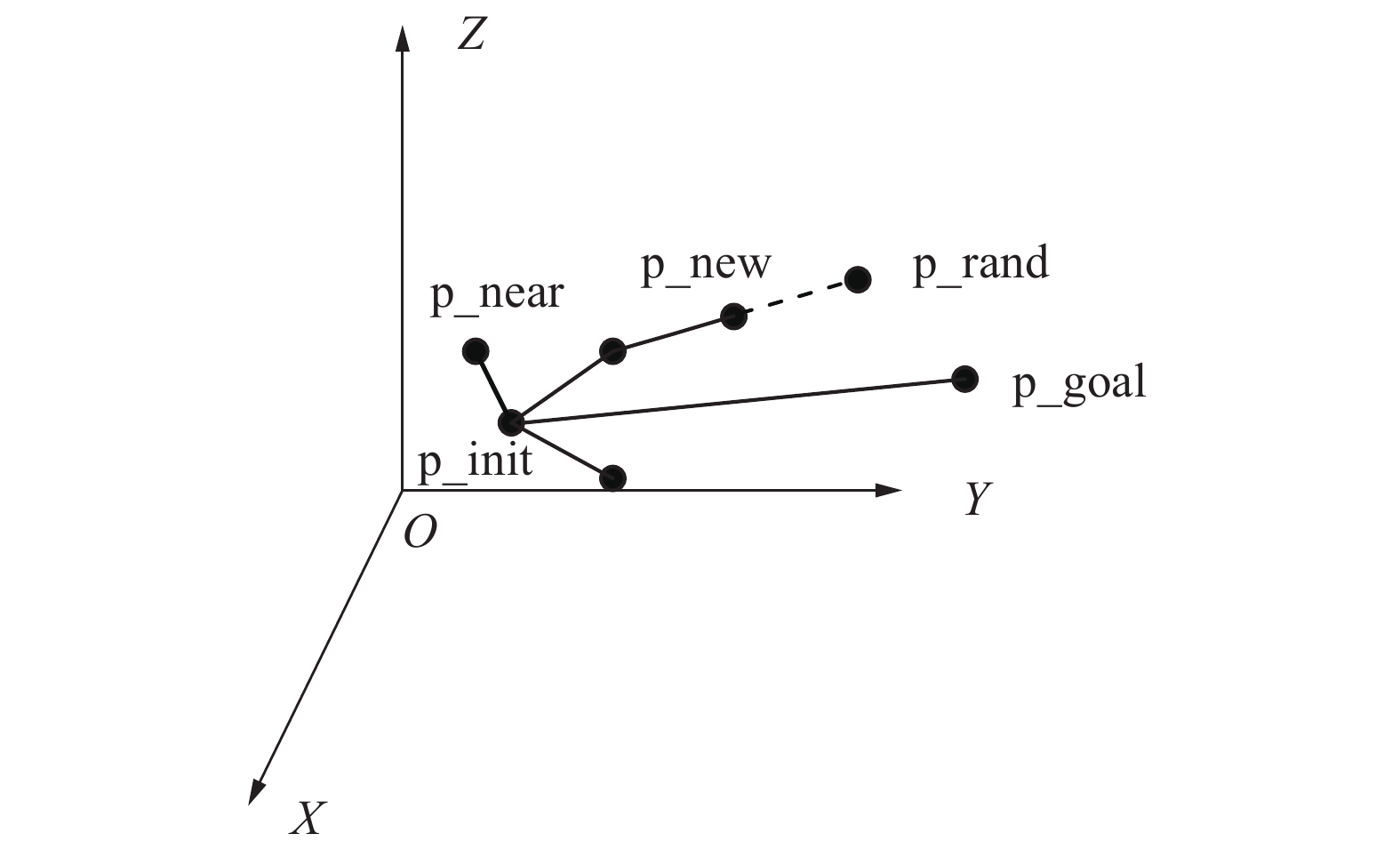图  1  三维RRT扩展过程
Fig.  1  3D RRT extend process

O是AUV所处的水下状态空间， $O \in W_n$。选择AUV的初始位置作为随机树的根节点p_init，根节点作为父节点在空间中随机采样得到p_rand，利用欧式距离在随机树上找距离p_rand最近的节点p_near，然后p_near以r步长向p_rand扩展得到新节点p_new，若p_near与p_new的连线通过了避碰检测，则将新节点p_new以及连线加入到随机树上。对上述过程重复进行，直到发现目标点。将随机树上的从根节点到目标点之间的树节点连接即为所规划的路径。

RRT算法的伪代码如下：

RRT algorithm

V={p_init}

for i=1 to I do

p_rand=Random()

p_near=Nearest(V, p_rand)

p_new=Extend(p_near, r, p_rand)

if Collision_free(p_new, p_near) then

Grow_tree(V, p_new)

end if

if ||p_new-p_goal|| $\leqslant$d_min then

return V

end if

end for

各函数解释如下：Random()为区域内随机选取一节点p_rand；Nearest()为在随机树上寻找距离p_rand最近的节点p_near；Extend()为从p_near向p_rand扩展步长r，得到新节点p_new。扩展关系式如下：

 \begin{aligned} {x_{\rm{new}}} = {x_{\rm{near}}} + r \cdot \sin \theta \cdot \cos \varphi \hfill \\ {y_{\rm{new}}} = {y_{\rm{near}}} + r \cdot \sin \theta \cdot \sin \varphi \hfill \\ {z_{\rm{new}}} = {z_{\rm{near}}} + r \cdot \cos \theta \quad\;\;\\ \end{aligned} (10)

Collision_free()为若p_new与p_near之间存在障碍物，重新选取节点。

从随机树的生长过程来看，一方面节点的选取具有盲目性，造成时间的延长，搜索效率降低；另一方面，RRT算法通常作为全局规划使用，不能直接应用到未知环境。为减少未知环境下的搜索时间以及提高搜索效率，本文将对以上不足做出改进。

##### 3.3.1   滚动规划

可以利用滚动规划解决三维水下环境复杂多变，很难获取完整的全局信息的问题。滚动规划主要分为预测、优化、反馈3 步。滚动过程中，以探测到的环境信息为基础，建立以AUV为中心的局部环境模型；将环境模型看作规划窗口，根据优化决策得到下一步的最优子目标点；在前往子目标点的过程中，根据探测到的新的环境信息，补充校正原来的模型滚动后的局部路径规划

本文滚动窗口以探测设备模型为基础建立，将探测环境窗口定义为滚动窗口，滚动窗口模型如图2所示。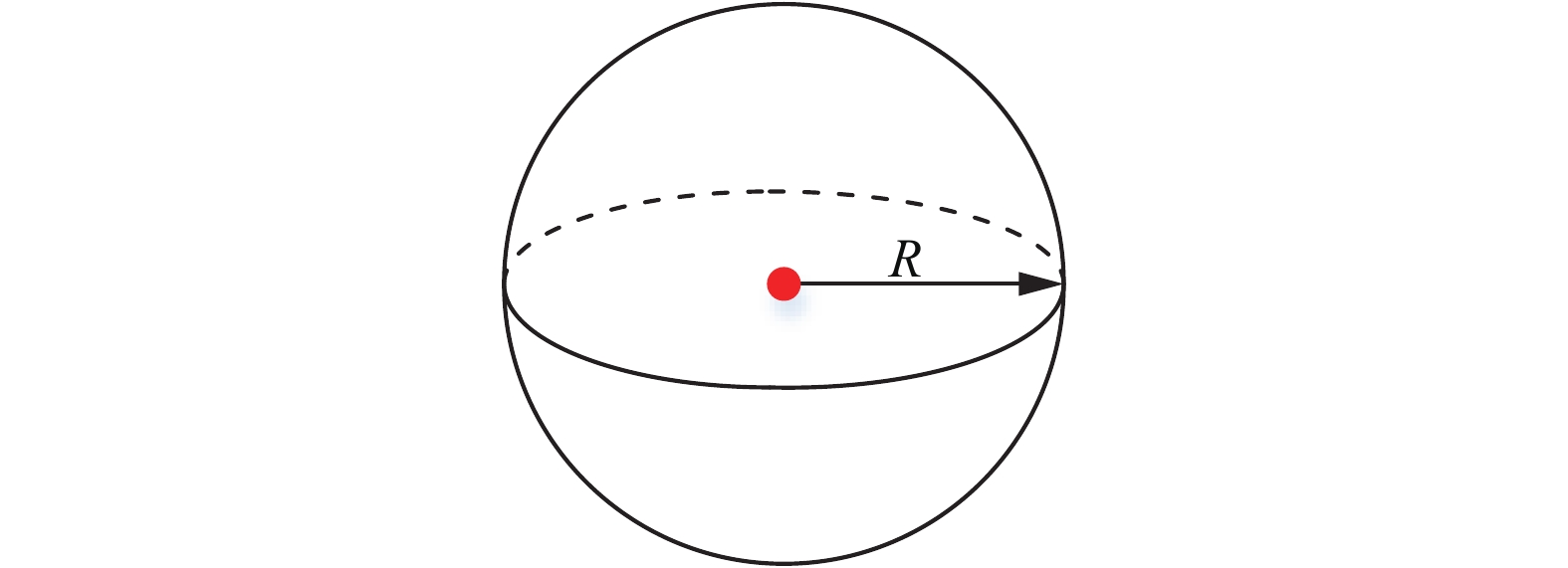图  2  滚动窗口
Fig.  2  Rolling window
##### 3.3.2   子目标点选取策略

记录t时刻航行器的所处位置为p_init(t)，将航行器探测设备能够探测到的环境窗口定义为当前滚动规划窗口

不同情形下的子目标选取策略不同，分别对以下3种情形进行讨论：1）目标在窗口内；2）窗口内不存在障碍物；3）窗口内存在障碍物。分别如图3~5

 $$\left\{ \begin{gathered} d({\rm{p\_init}}(t),{\rm{p\_goal}}(t)) \leqslant R \hfill \\ {\rm{p\_temp}}(t) = {\rm{p\_goal}}(t) \hfill \\ \end{gathered} \right.$$ (11)
 $$\left\{ \begin{gathered} d({\rm{p\_init}}(t),{\rm{p\_temp}}(t)) = R \hfill \\ {\rm{p\_temp}}(t) = f(\min (d({\rm{p\_init}}(t),{\rm{p\_goal}}(t)))) \hfill \\ \end{gathered} \right.$$ (12)
 $$\left\{ \begin{gathered} {\rm{p\_new}}(t) = {\rm{Extend}}({\rm{p\_init}}(t),r) \hfill \\ d({\rm{p\_new}}(t),{\rm{p\_temp}}(t)) = R \hfill \\ {\rm{p\_temp}}(t) = f(\min (d({\rm{p\_new}}(t),{\rm{p\_goal}}(t)))) \hfill \\ \end{gathered} \right.$$ (13)

分别根据情况采用式（11）~（13）确定子目标点p_temp(t)图3~5分别对应式（11）~（13）。

##### 3.3.3   反向节点裁剪规则

为降低p_rand的随机性，引入反向节点裁剪规则，即控制(p_init, p_new)向量同(p_init, p_goal)向量间夹角为锐角，避免反向选取节点。三维环境下，为控制向量夹角，分别从声呐水平探测开角和纵深探测开角考虑，故将向量分别投影到xoy轴和yoz轴，得到投影角µ1µ2，如图6图7所示。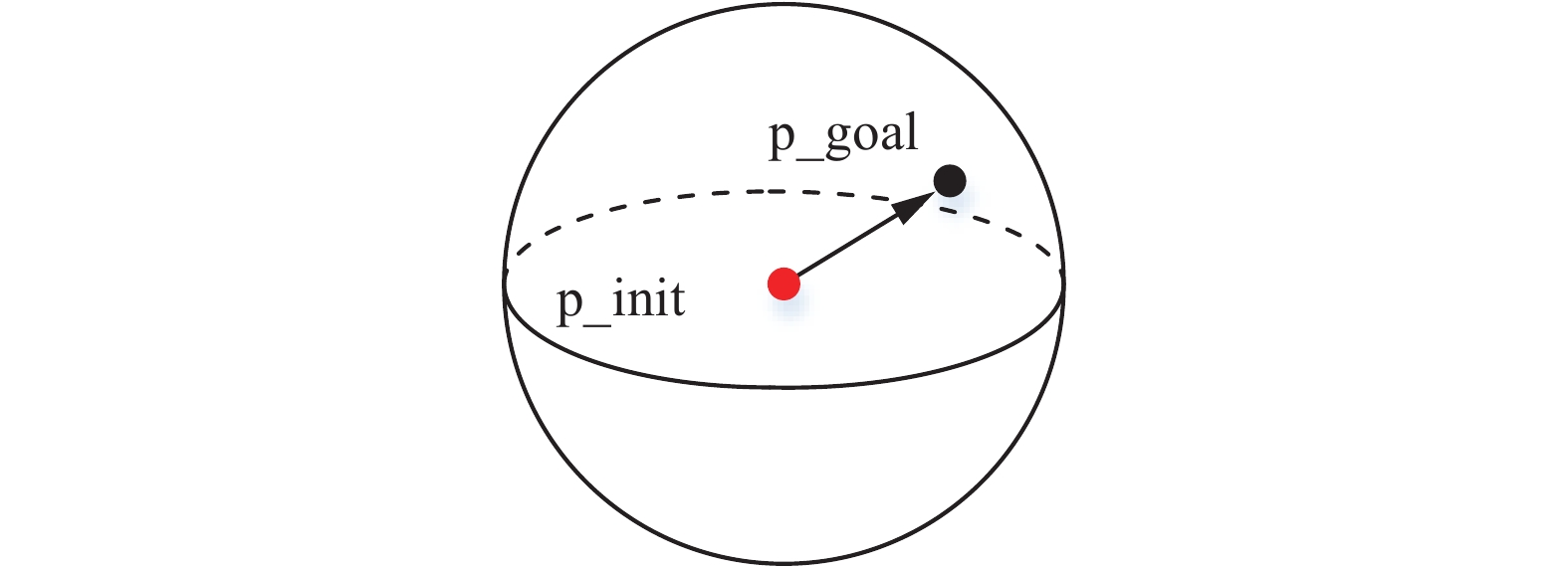图  3  目标在窗口内
Fig.  3  Target in window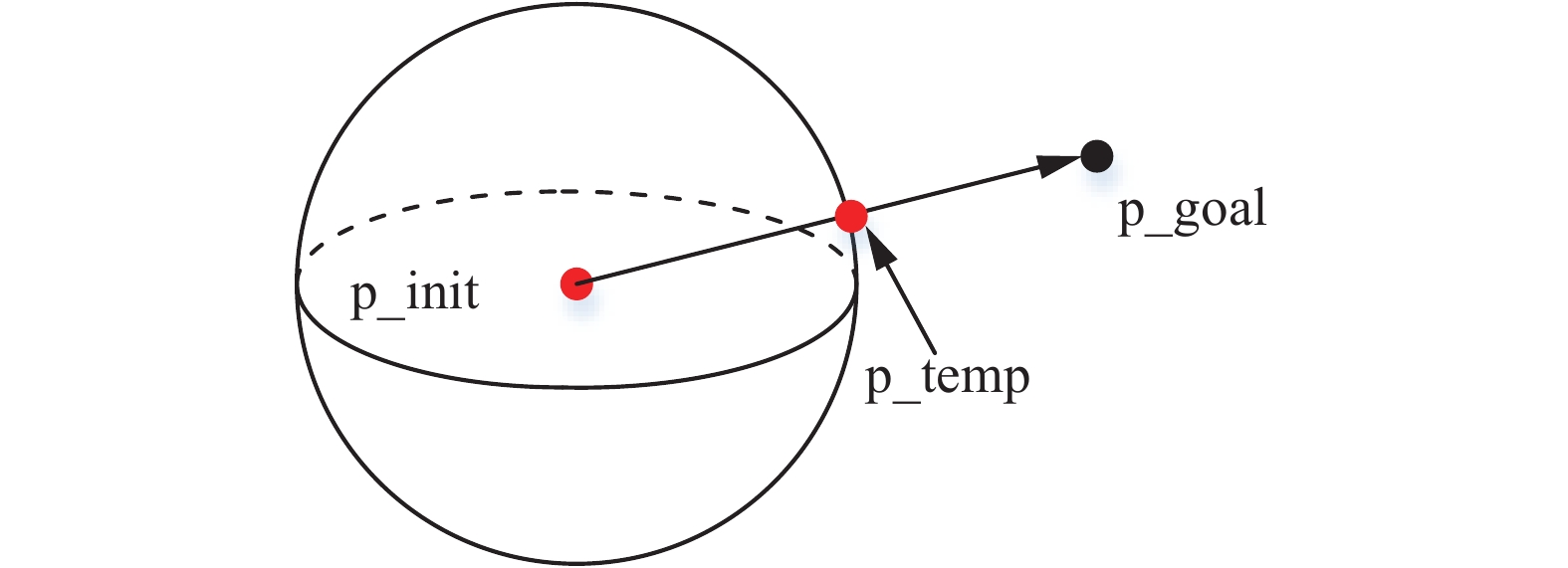图  4  窗口内不存在障碍物
Fig.  4  No obstacles in the window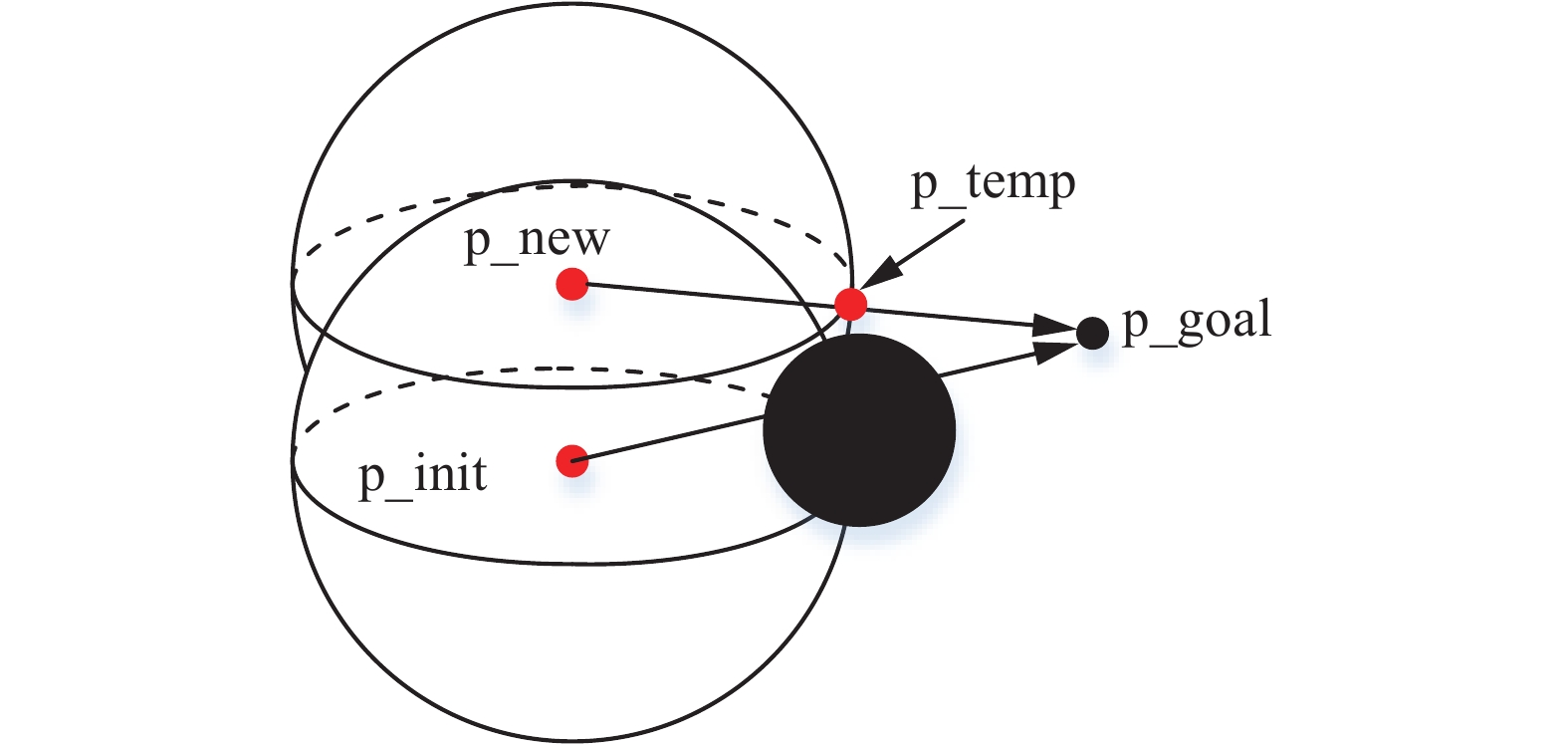图  5  窗口内存在障碍物
Fig.  5  Obstacles in the window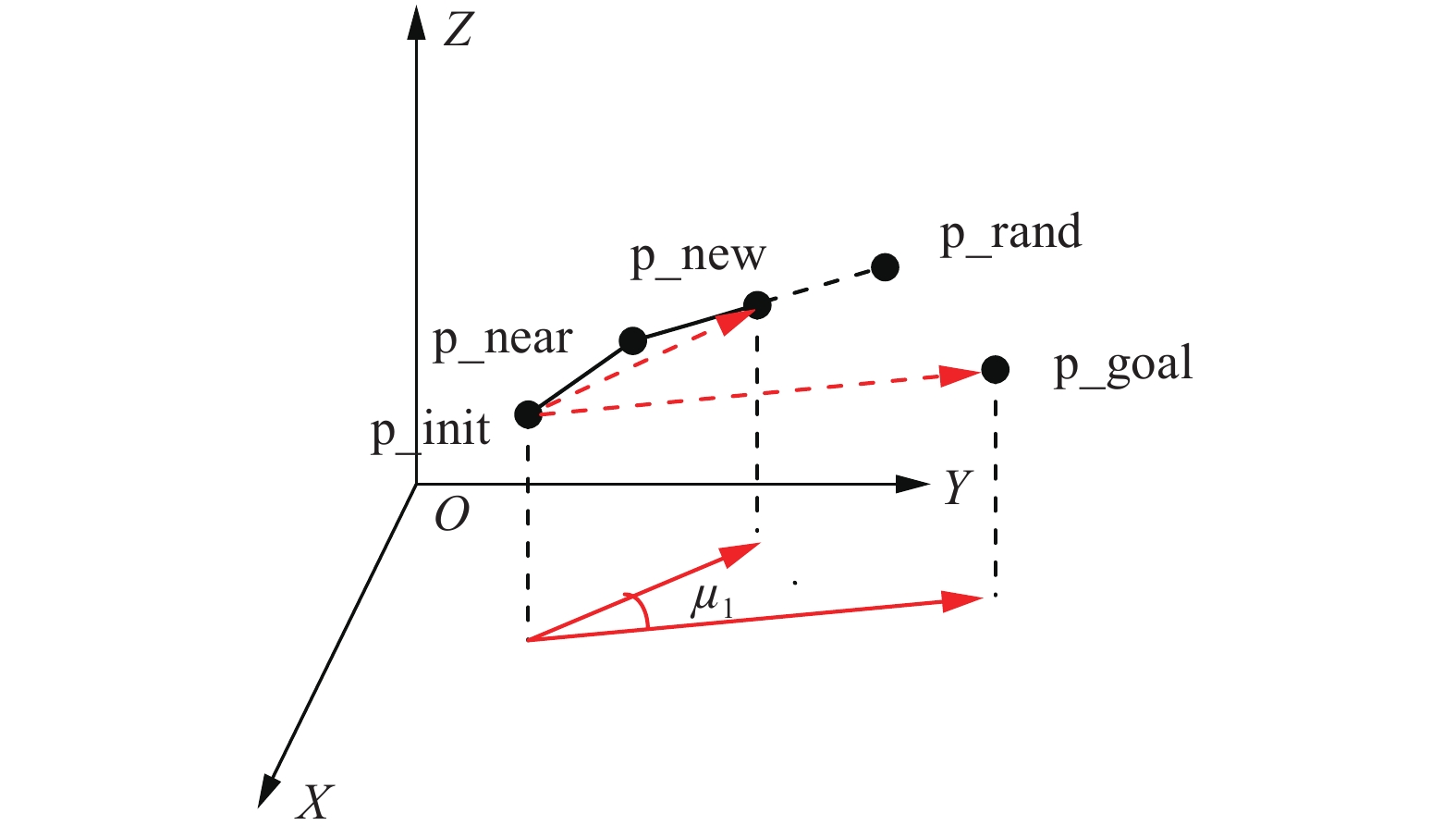图  6  XOY平面投影
Fig.  6  Projected on theXOY plane

为验证引入滚动思想的三维RRT算法的有效性，设计了 RRT 算法与滚动 RRT 算法的对比仿真实验。仿真环境800 m×800 m×800 m，AUV的探测半径为 100 m，起始点坐标为（0,0,0），目标点坐标为（750,750,750），随机树的扩展步长为80。其中随机节点以及扩展树枝均为蓝色，起点用红色标记，目标点以及子目标点用绿色表示。灰色透明球体分别表示滚动窗口以及障碍物。如图8 为 RRT 算法规划的过程，红色实线为最终路径。图9图10 分别为改进滚动 RRT 算法规划过程的两个视角，红色实线为最终路径。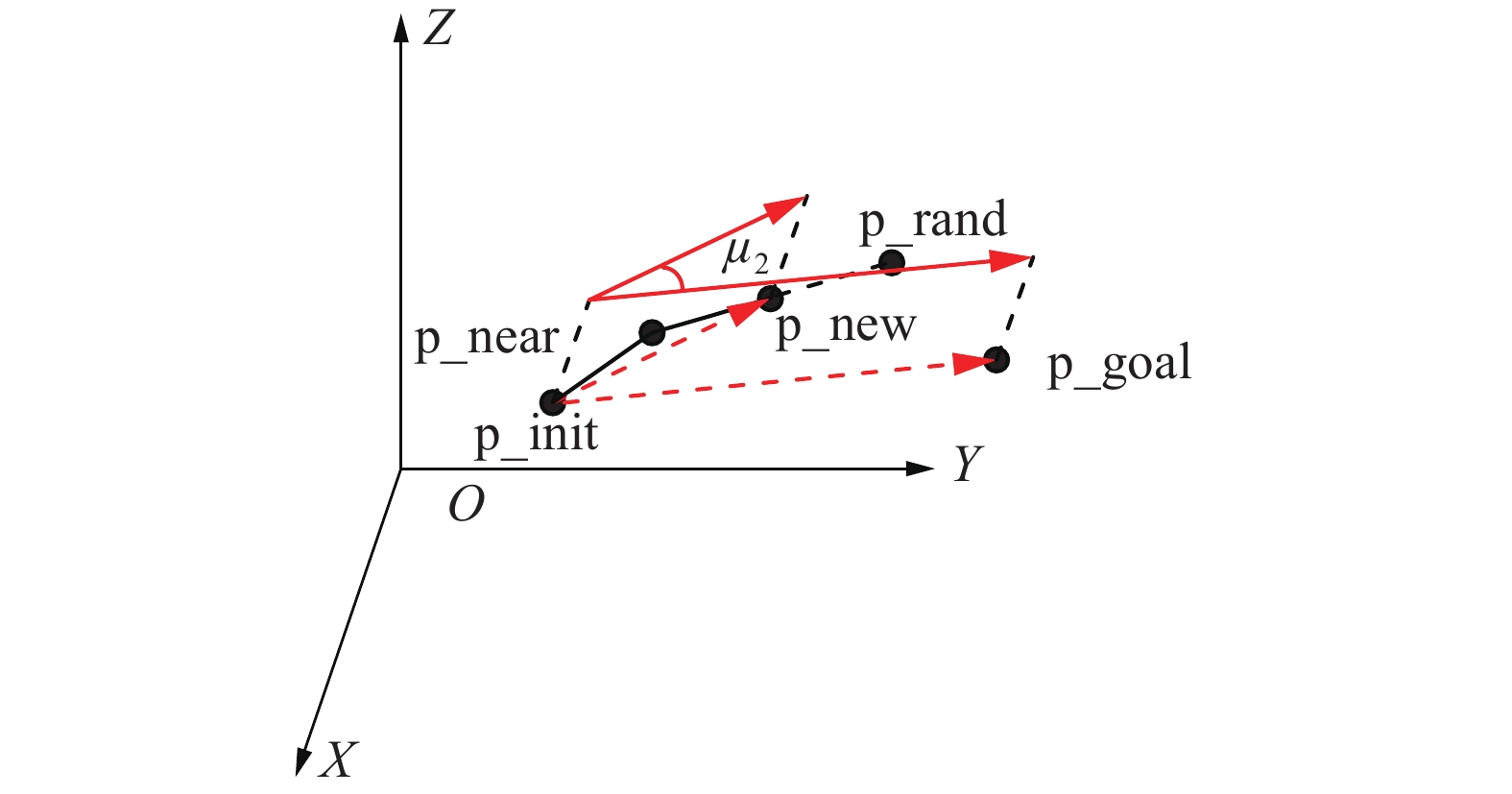图  7  YOZ平面投影
Fig.  7  Projected on the YOZ plane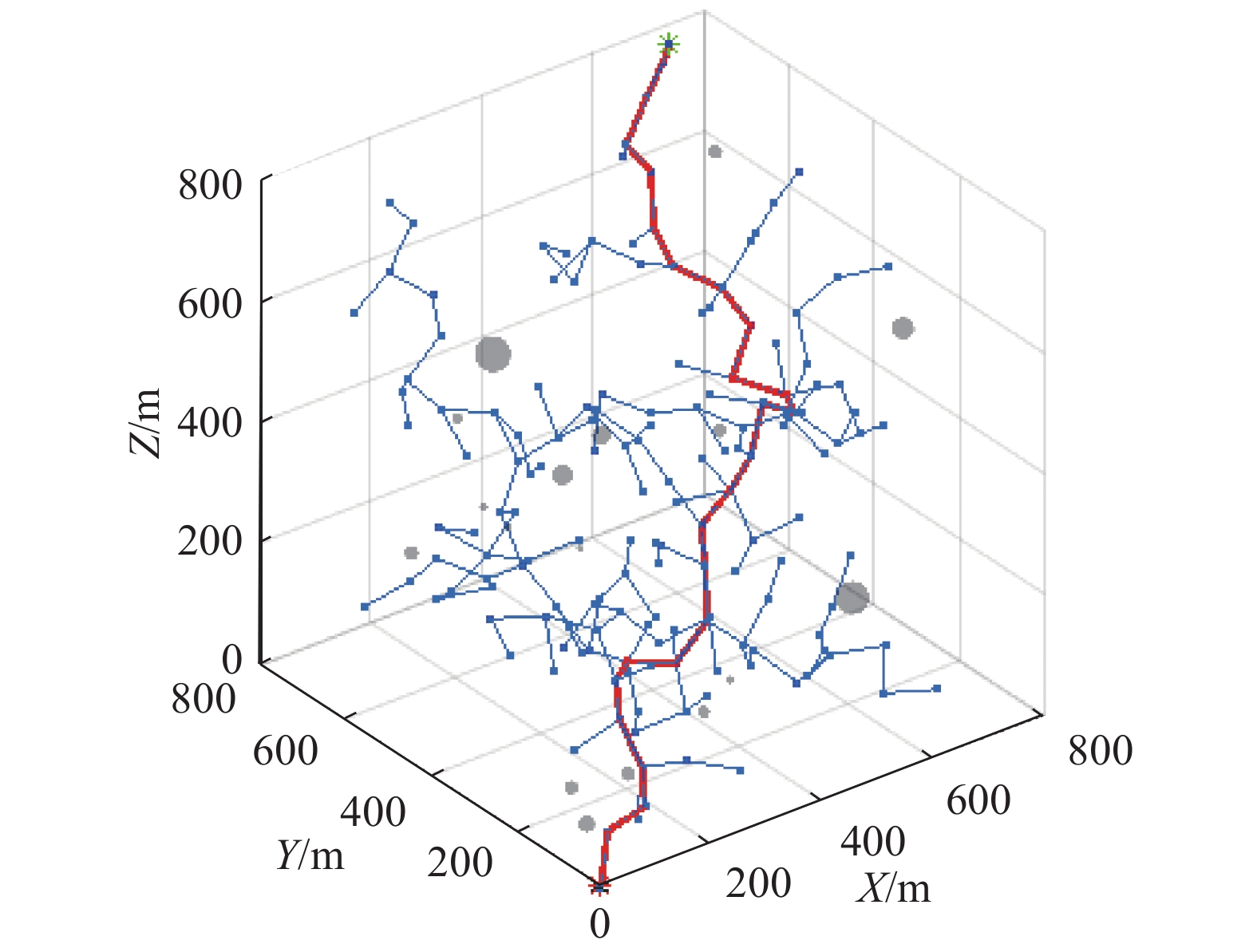图  8  RRT算法
Fig.  8  RRT algorithm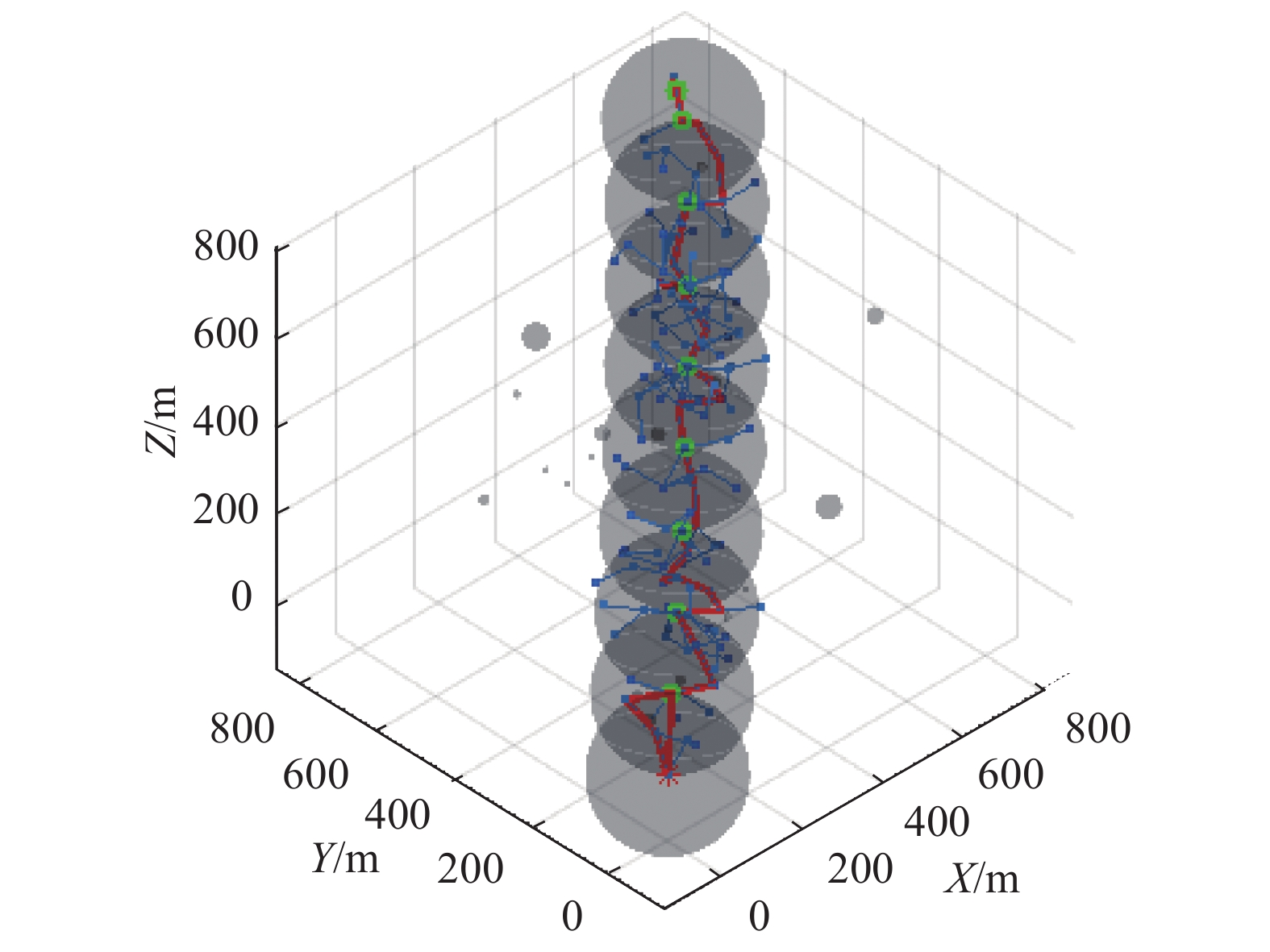图  9  改进滚动RRT算法（角度1）
Fig.  9  Improved rolling RRT algorithm(point 1)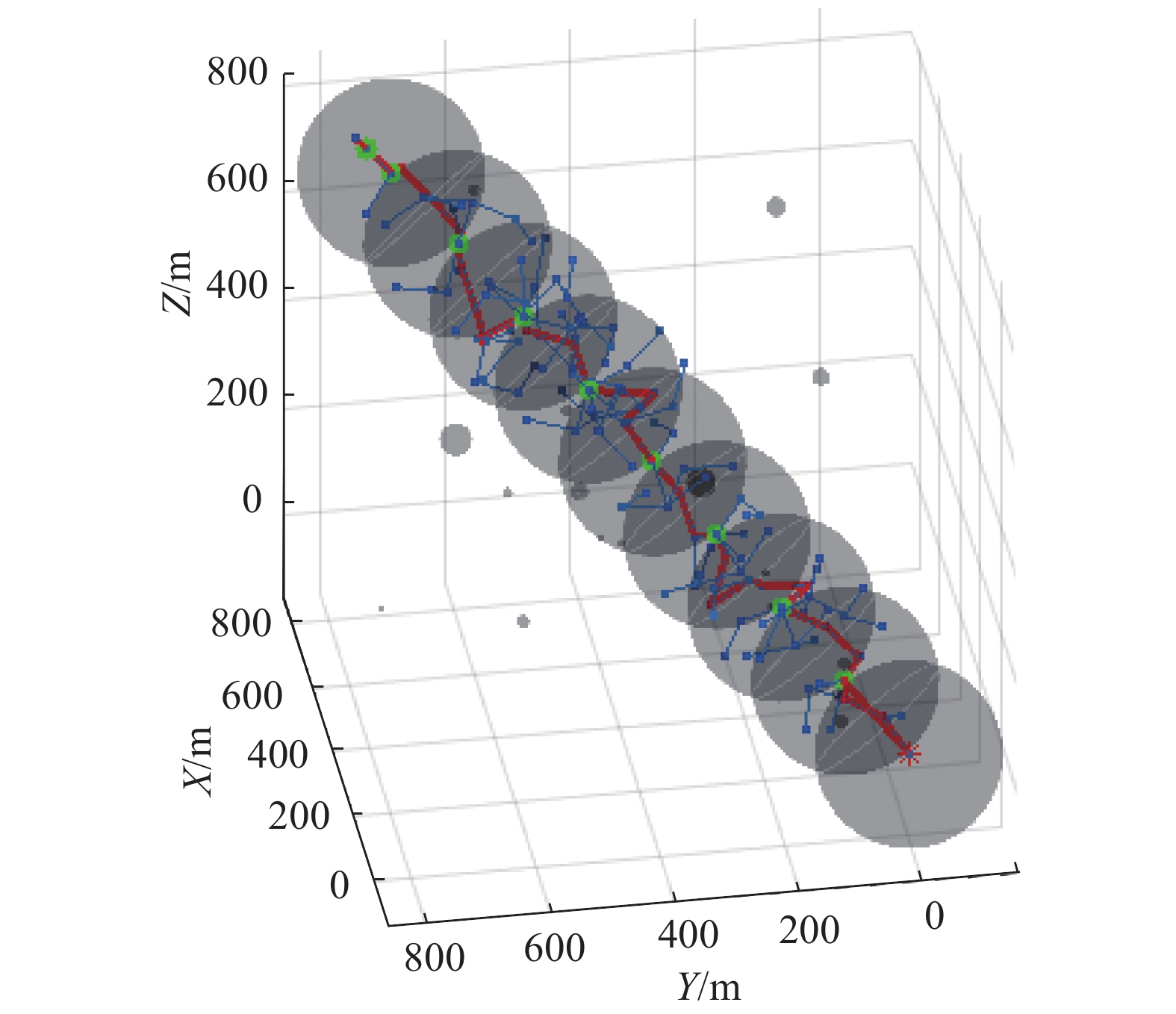图  10  改进滚动RRT算法（角度2）
Fig.  10  Improved rolling RRT algorithm(point 2)

选取20次实验中具有代表性的5组数据，对比两种算法的路径规划结果，从表1可以看到相对于传统RRT，引入滚动思想的RRT算法通过限制节点的选取范围以及树枝的长度大大减少了节点数量，缩短了规划时间，路径规划效率有了很大的提升。

表  1  RRT同改进滚动RRT对比
Table  1  Comparison of RRT and Improved rolling RRT
 算法 节点/个 路径/m RRT 233 2800 234 2093 151 3018 328 4416 267 2517 改进滚动RRT 114 1897 110 1812 41 2282 27 2485 87 1688

本节仿真设置搜索区域实际大小为800 m×800 m×200 m，声呐传感器的探测半径R为 100 m，检测概率 $P_d=0.9$，虚警概率 $P_f=0.1$。将搜索区域栅格化。仿真过程中将按障碍物的最大轮廓线膨化为球形，从而实现最大程度避碰，并将 AUV和目标看作质点。

在 AUV航行过程中，AUV航速设置为2 m/s。本文采用步数为算法计量单位，考虑 AUV 的速度，设定 AUV 每航行2 m为一步，每步所用时间为1 s。

AUV 的初始坐标为(0,0,0)，设置静目标11个，目标用五角星表示，球体障碍物7个，用黑色球体表示，如图11为搜索的初始状态。红色实线代表AUV的实时搜索路径。如图12 为RRT 算法目标搜索算法，红色实线为搜索路径。图13为改进滚动 RRT 目标搜索算法，红色实线为搜索路径。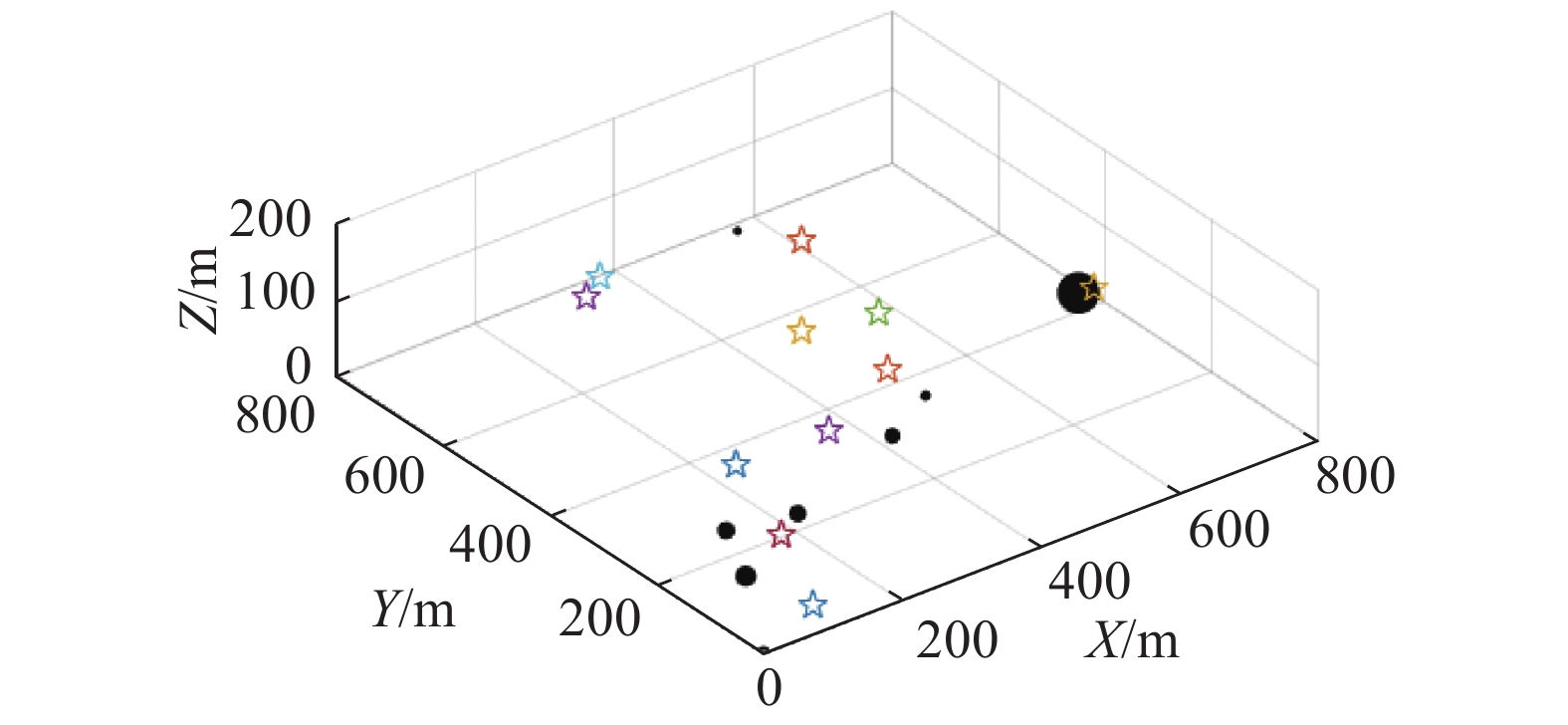图  11  初始状态
Fig.  11  Initial state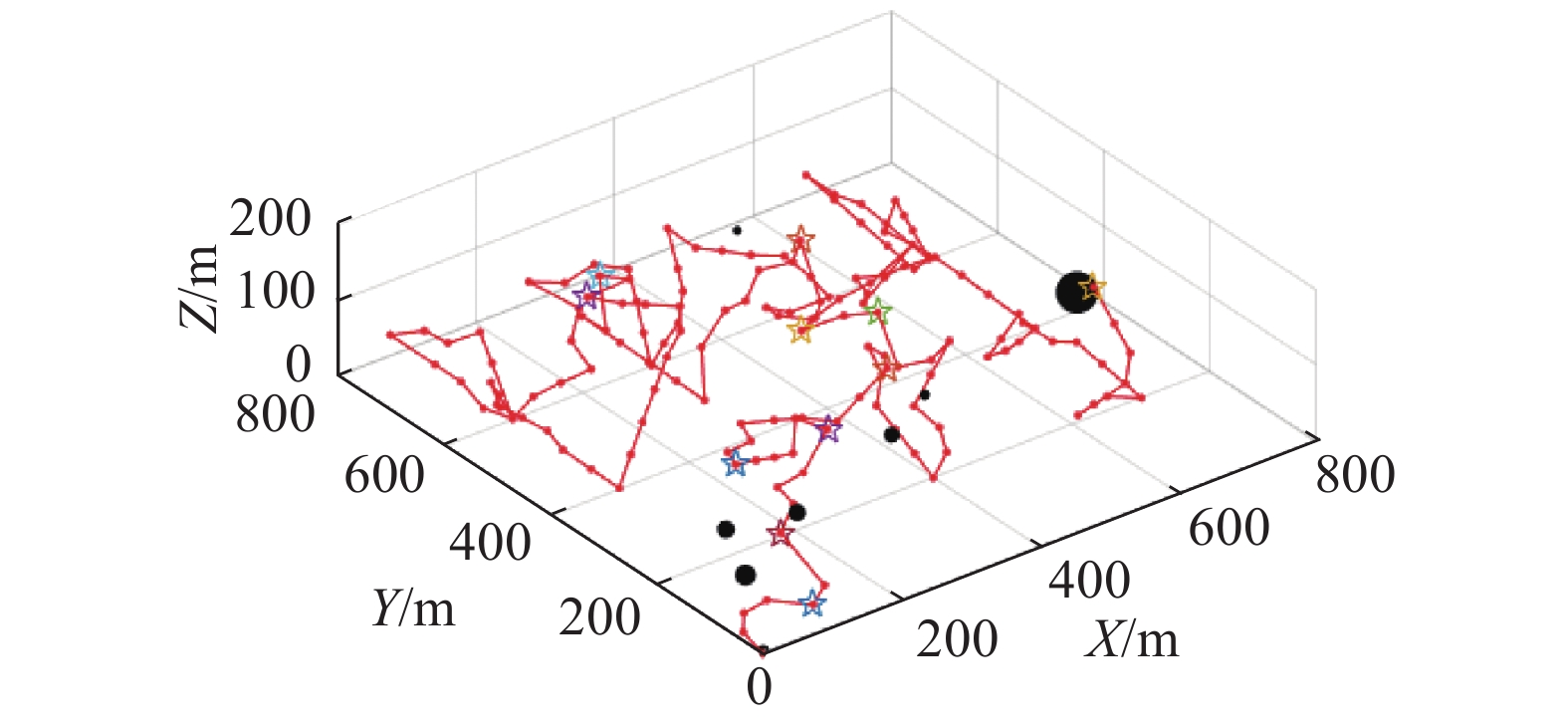图  12  RRT目标搜索算法
Fig.  12  Target search based on RRT algorithm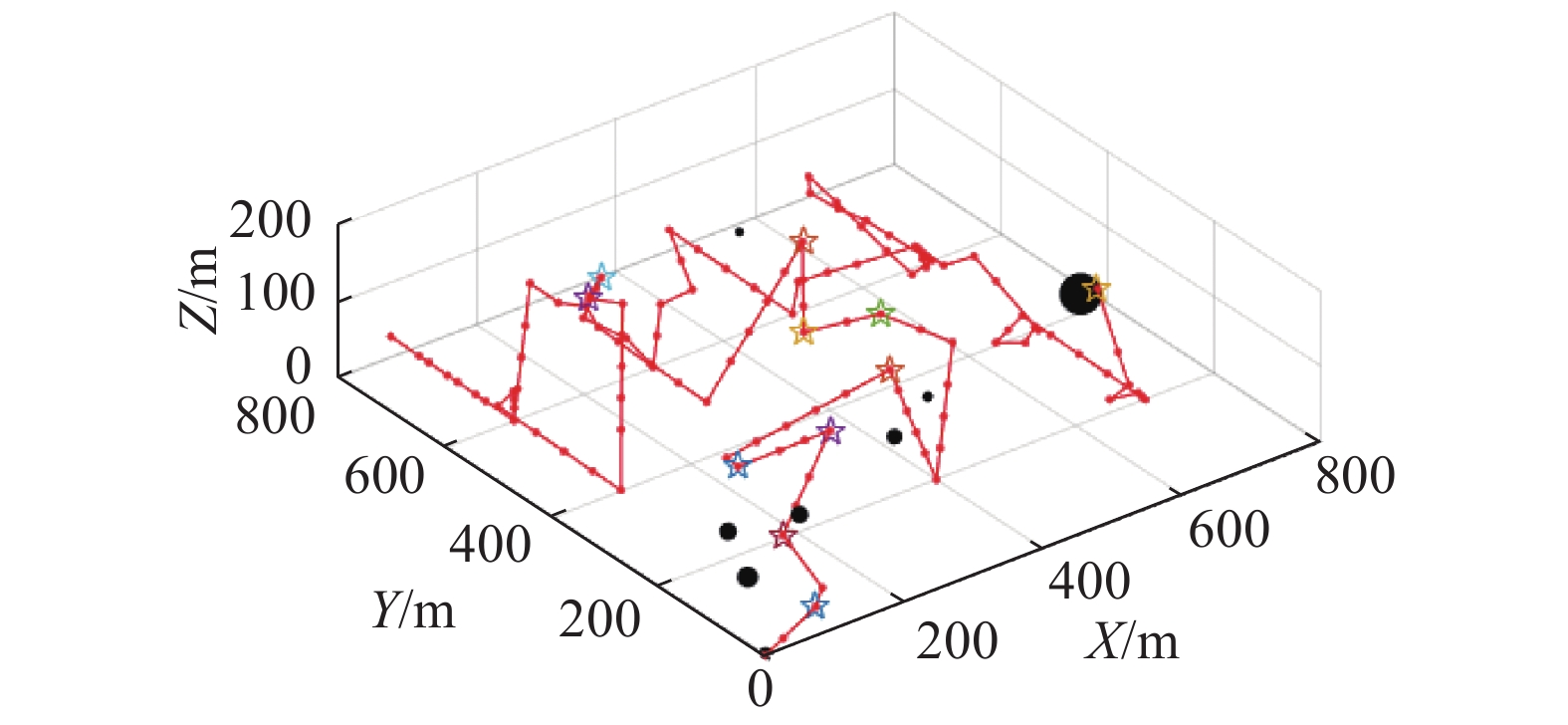图  13  改进滚动RRT目标搜索算法
Fig.  13  Target search based on improved rolling RRT algorithm

因为搜索决策函数一致，从图12图13中看出，RRT目标搜索算法与滚动RRT搜索算法的搜索路径大致相同，但从表2中可以看出，应用滚动RRT算法的搜索时间明显少于RRT算法，搜索速度有了很大的提升。

表  2  RRT搜索同改进滚动RRT搜索对比
Table  2  Comparison of RRT and improved rolling RRT
 算法 时间/s 路径/m RRT 30681 61367 改进滚动RRT 21987 43969

本文提出基于滚动RRT算法的三维目标搜索算法，算法从决策点规划角度入手，利用RRT算法快速性的特点提高整体环境的搜索速度。滚动规划结合子目标点选取策略，将全局路径规划细分为一步步的局部路径规划，使得 RRT 算法应用到未知环境；其次，利用搜索决策函数求出决策点后，应用改进滚动RRT算法实时规划路径；最后通过仿真验证了算法的高效性。另外，多次仿真过程中，出现了AUV对同一区域多次搜索并陷入当前搜索环境的情况，避免区域重复搜索方面并没有很好的实现；在决策点路径规划时，多次出现规划时间过长的情况；实际海底环境存在非常多的障碍物或是形状复杂的障碍物，单纯利用文章中提出的改进算法，可能会导致失败的情况发生，因此要通过实际的水下试验来验证以及优化；针对这三类问题仍有必要继续深入研究。

• 图  1   三维RRT扩展过程

Fig.  1   3D RRT extend process

图  2   滚动窗口

Fig.  2   Rolling window

图  3   目标在窗口内

Fig.  3   Target in window

图  4   窗口内不存在障碍物

Fig.  4   No obstacles in the window

图  5   窗口内存在障碍物

Fig.  5   Obstacles in the window

图  6   XOY平面投影

Fig.  6   Projected on theXOY plane

图  7   YOZ平面投影

Fig.  7   Projected on the YOZ plane

图  8   RRT算法

Fig.  8   RRT algorithm

图  9   改进滚动RRT算法（角度1）

Fig.  9   Improved rolling RRT algorithm(point 1)

图  10   改进滚动RRT算法（角度2）

Fig.  10   Improved rolling RRT algorithm(point 2)

图  11   初始状态

Fig.  11   Initial state

图  12   RRT目标搜索算法

Fig.  12   Target search based on RRT algorithm

图  13   改进滚动RRT目标搜索算法

Fig.  13   Target search based on improved rolling RRT algorithm

表  1   RRT同改进滚动RRT对比

Table  1   Comparison of RRT and Improved rolling RRT

 算法 节点/个 路径/m RRT 233 2800 234 2093 151 3018 328 4416 267 2517 改进滚动RRT 114 1897 110 1812 41 2282 27 2485 87 1688

表  2   RRT搜索同改进滚动RRT搜索对比

Table  2   Comparison of RRT and improved rolling RRT

 算法 时间/s 路径/m RRT 30681 61367 改进滚动RRT 21987 43969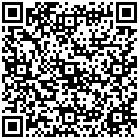点击查看大图

##### 出版历程
• 收稿日期:  2021-05-13
• 网络出版日期:  2021-10-19

### 目录/

• 分享
•用微信扫码二维码

分享至好友和朋友圈